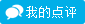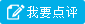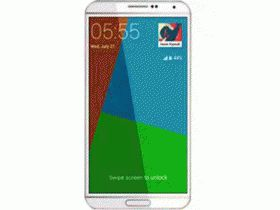##三星 GALAXY Note 4点评42人(26%)

41人(26%)

39人(24%)

38人(24%)

5.0

5.0

5.0

5.0

3.2

2.8

3.5

3.2

0.5

0.1

2.9

1.0
• 电池不经用
• 对不起这个品牌
• 触屏响应迟钝
• 流畅度一般

5.0

5.0

5.0

5.0
• 分辨率高
• 大气
• 外观漂亮
• 当平板玩
• 屏幕适中
• 系统流畅
• 拍照神器
• 游戏神器
• 握感舒适
• 喜欢这品牌
• 色彩鲜明
• 开机很快
• 话音清晰
• 质感精致
• 色彩明亮
• 电池扛用
• 价格合理

3.0

2.9

5.0

2.1

5.0

3.0

4.5

0.5
• 价格虚高
• 性价比低

3.8

3.9

3.0

3.1
• 老人机器

0.5

0.4

0.3

0.4
• 喂喂靠

3.6

2.5

4.0

4.0
• 大气
• 外观漂亮
• 自拍神器
• 屏幕巨大
• 音乐神器
• 当平板玩
• 电池不经用
• 价格虚高
• 系统流畅
• 拍照神器
• 影视神器
• 握感舒适
• 梦想拥有
• 喜欢这品牌
• 色彩鲜明
• 话音清晰
• 质感精致
• 商务范
• 分辨率一般
• 机脆脆
• 开机一般
• 不怕水
• 触感粗糙

4.5

2.5

4.5

4.5
• 大气
• 屏幕巨大
• 当平板玩
• 电池不经用
• 价格虚高
• 系统流畅
• 拍照神器
• 自拍一般
• 影视神器
• 梦想拥有
• 色彩鲜明
• 喂喂靠
• 商务范
• 机脆脆
• 开机一般

4.7

1.9

5.0

3.2
• 大气
• 外观漂亮
• 音乐神器
• 当平板玩
• 电池不经用
• 价格虚高
• 系统流畅
• 拍照神器
• 自拍一般
• 影视神器
• 握感舒适
• 话音清晰
• 性价比低
• 商务范
• 开机一般
• 不符我的个性
• 视频播放跳帧
• 太滑溜了

3.0

2.0

3.0

3.0
• 分辨率高
• 屏幕巨大
• 系统流畅
• 拍照神器
• 梦想拥有
• 色彩鲜明
• 话音清晰
• 电池扛用

0.0

0.0

0.0

0.0

4.8

0.1

4.8

4.4
• 唯美
• 大气
• 外观漂亮
• 土豪
• 音乐神器
• 电池不经用
• 价格虚高
• 握感舒适
• 喜欢这品牌
• 色彩鲜明
• 话音清晰
• 大小适中
• 商务范
• 分辨率一般
• 拍照一般
• 开机一般
• 自拍很差
• 不怕水
• 时尚女魔头

3.7

0.7

4.4

1.5

0.0

0.0

0.0

0.0

5.0

5.0

5.0

5.0
• 外观漂亮
• 屏幕巨大
• 音乐神器
• 当平板玩
• 电池不经用
• 价格虚高
• 系统流畅
• 自拍一般
• 握感舒适
• 喜欢这品牌
• 色彩鲜明
• 开机很快
• 喂喂靠
• 商务范
• 分辨率一般
• 拍照一般
• 能砸核桃

4.7

4.5

5.0

5.0
• 适合女生
• 电池不经用
• 质感精致
• 不怕水
• 时尚女魔头

5.0

4.0

4.6

4.5
• 简约
• 分辨率高
• 大气
• 外观漂亮
• 屏幕巨大
• 价格虚高
• 游戏神器
• 梦想拥有
• 色彩鲜明
• 质感精致
• 商务范

5.0

0.2

5.0

5.0
• 性价比高

4.5

4.0

4.5

4.5
• 简约
• 分辨率高
• 唯美
• 大气
• 外观漂亮
• 价格虚高
• 屏幕适中
• 系统流畅
• 拍照神器
• 自拍一般
• 影视神器
• 握感舒适
• 喜欢这品牌
• 色彩鲜明
• 话音清晰
• 大小适中
• 质感精致
• 开机一般

5.0

0.4

5.0

2.4
• 外观漂亮
• 喜欢这品牌

4.3

2.5

4.5

2.5
• 分辨率高
• 山寨
• 屏幕巨大
• 电池不经用
• 色彩鲜明
• 开机很快
• 对不起这个品牌
• 性价比低
• 拍照很差
• 分辨率一般
• 户外机
• 开机一般
• 自拍很差
• 能砸核桃
• 适合学生

5.0

2.7

5.0

5.0
• 简约
• 分辨率高
• 大气
• 音乐神器
• 当平板玩
• 电池不经用
• 价格虚高
• 屏幕适中
• 拍照神器
• 自拍一般
• 握感舒适
• 喜欢这品牌
• 色彩鲜明
• 开机很快
• 话音清晰
• 商务范
• 备用机
• 流畅度一般
• 不怕水
• 能砸核桃
• 游戏卡顿

4.4

1.3

4.4

4.5
• 简约
• 分辨率高
• 老人机
• 大气
• 电池不经用
• 价格虚高
• 屏幕适中
• 自拍一般
• 影视神器
• 握感舒适
• 喜欢这品牌
• 开机很快
• 话音清晰
• 大小适中
• 色彩明亮
• 拍照一般
• 机脆脆
• 户外机
• 流畅度一般

1.7

1.1

1.2

0.9
• 适合女生
• 电池不经用
• 经常误操作
• 开机一般
• 适合学生
• 游戏卡顿

3.3

0.3

4.3

0.2
• 分辨率高
• 老人机
• 电池不经用
• 价格虚高
• 拍照神器
• 自拍一般
• 色彩鲜明
• 话音清晰
• 大小适中
• 质感精致
• 对不起这个品牌
• 经常误操作
• 开机一般
• 流畅度一般
• 不符我的个性

4.0

0.6

0.9

1.8
• 电池不经用
• 价格虚高
• 屏幕适中
• 拍照一般
• 开机一般
• 游戏卡顿

3.8

3.0

4.0

3.0
• 山寨
• 适合女生
• 屏幕巨大
• 电池不经用
• 价格虚高
• 自拍一般
• 影视神器
• 握感舒适
• 色彩鲜明
• 话音清晰
• 大小适中
• 分辨率一般
• 拍照一般
• 开机一般
• 触屏响应迟钝
• 备用机
• 能砸核桃

1.4

1.9

1.4

1.8
• 大气
• 音乐神器
• 电池不经用
• 价格虚高
• 握感舒适
• 喜欢这品牌
• 大小适中
• 色彩很差
• 喂喂靠
• 分辨率一般
• 拍照一般
• 机脆脆
• 开机一般
• 流畅度一般
• 自拍很差

5.0

4.8

4.8

4.8
• 大气
• 屏幕巨大
• 电池不经用
• 价格虚高
• 自拍一般
• 影视神器
• 握感舒适
• 梦想拥有
• 色彩鲜明
• 大小适中
• 喂喂靠
• 商务范
• 分辨率一般
• 拍照一般
• 机脆脆
• 开机一般
• 触屏响应迟钝

5.0

5.0

5.0

5.0
• 精巧
• 简约
• 分辨率高
• 唯美
• 性价比高
• 大气
• 外观漂亮
• 自拍神器
• 音乐神器
• 电池不经用
• 屏幕适中
• 系统流畅
• 拍照神器
• 游戏神器
• 影视神器
• 握感舒适
• 开机很快
• 话音清晰
• 大小适中
• 质感精致
• 商务范
• 色彩明亮

4.0

2.4

3.1

3.3

4.5

4.0

4.5

4.5
• 分辨率高
• 大气
• 外观漂亮
• 电池不经用
• 屏幕适中
• 系统流畅
• 拍照神器
• 游戏神器
• 色彩鲜明
• 话音清晰
• 大小适中
• 质感精致
• 性价比低
• 开机一般
• 备用机

1.0

2.0

1.5

1.0
• 简约
• 老人机
• 适合女生
• 电池不经用
• 价格虚高
• 屏幕适中
• 自拍一般
• 对不起这个品牌
• 色彩很差
• 喂喂靠
• 分辨率一般
• 色彩明亮
• 拍照一般
• 开机一般
• 流畅度一般
• 自拍很差
• 不符我的个性
• 游戏卡顿

1.6

1.2

1.4

0.8
• 分辨率高
• 老人机
• 土豪
• 屏幕巨大
• 当平板玩
• 电池不经用
• 拍照神器
• 开机很快
• 喂喂靠
• 机脆脆
• 备用机
• 时尚女魔头

5.0

5.0

5.0

5.0
• 喜欢这品牌
• 简约
• 分辨率高
• 大气
• 外观漂亮
• 适合女生
• 自拍神器
• 屏幕巨大
• 音乐神器
• 系统流畅
• 拍照神器
• 游戏神器
• 影视神器
• 梦想拥有
• 色彩鲜明
• 开机很快
• 话音清晰
• 大小适中
• 质感精致
• 电池扛用
• 价格合理
• 时尚女魔头
• 能砸核桃
• 适合学生

5.0

5.0

5.0

5.0
• 简约
• 分辨率高
• 唯美
• 大气
• 外观漂亮
• 自拍神器
• 屏幕巨大
• 系统流畅
• 拍照神器
• 游戏神器
• 梦想拥有
• 色彩鲜明
• 开机很快
• 话音清晰
• 大小适中
• 质感精致
• 商务范
• 电池扛用
• 价格合理
• 能砸核桃
• 适合学生

5.0

5.0

5.0

5.0
• 分辨率高
• 大气
• 外观漂亮
• 自拍神器
• 屏幕巨大
• 系统流畅
• 拍照神器
• 游戏神器
• 梦想拥有
• 色彩鲜明
• 开机很快
• 话音清晰
• 大小适中
• 质感精致
• 商务范
• 电池扛用
• 价格合理
• 能砸核桃

5.0

5.0

5.0

5.0

5.0

5.0

5.0

5.0
• 分辨率高
• 唯美
• 大气
• 自拍神器
• 屏幕巨大
• 系统流畅
• 拍照神器
• 游戏神器
• 梦想拥有
• 色彩鲜明
• 开机很快
• 话音清晰
• 大小适中
• 质感精致
• 商务范
• 电池扛用
• 户外机
• 价格合理
• 能砸核桃

5.0

5.0

5.0

5.0
• 分辨率高
• 大气
• 外观漂亮
• 适合女生
• 自拍神器
• 屏幕巨大
• 系统流畅
• 拍照神器
• 游戏神器
• 梦想拥有
• 色彩鲜明
• 开机很快
• 话音清晰
• 大小适中
• 质感精致
• 电池扛用
• 价格合理
• 时尚女魔头
• 能砸核桃
• 适合学生

5.0

5.0

5.0

5.0
• 分辨率高
• 外观漂亮
• 自拍神器
• 屏幕巨大
• 系统流畅
• 拍照神器
• 游戏神器
• 梦想拥有
• 色彩鲜明
• 开机很快
• 话音清晰
• 大小适中
• 质感精致
• 商务范
• 电池扛用
• 户外机
• 价格合理
• 能砸核桃
• 适合学生

5.0

5.0

5.0

5.0
• 分辨率高
• 大气
• 外观漂亮
• 自拍神器
• 土豪
• 屏幕巨大
• 系统流畅
• 拍照神器
• 游戏神器
• 梦想拥有
• 色彩鲜明
• 开机很快
• 话音清晰
• 大小适中
• 质感精致
• 商务范
• 电池扛用
• 价格合理
• 能砸核桃

5.0

5.0

5.0

5.0
• 简约
• 分辨率高
• 唯美
• 大气
• 外观漂亮
• 适合女生
• 自拍神器
• 土豪
• 屏幕巨大
• 系统流畅
• 拍照神器
• 游戏神器
• 梦想拥有
• 色彩鲜明
• 开机很快
• 话音清晰
• 大小适中
• 质感精致
• 商务范
• 电池扛用
• 户外机
• 价格合理
• 时尚女魔头
• 能砸核桃
• 适合学生

5.0

5.0

5.0

5.0
• 简约
• 分辨率高
• 唯美
• 大气
• 外观漂亮
• 自拍神器
• 土豪
• 屏幕巨大
• 系统流畅
• 拍照神器
• 游戏神器
• 梦想拥有
• 色彩鲜明
• 开机很快
• 话音清晰
• 大小适中
• 质感精致
• 商务范
• 电池扛用
• 户外机
• 价格合理
• 能砸核桃
• 适合学生

5.0

5.0

5.0

5.0
• 分辨率高
• 外观漂亮
• 适合女生
• 自拍神器
• 屏幕巨大
• 系统流畅
• 拍照神器
• 游戏神器
• 梦想拥有
• 色彩鲜明
• 开机很快
• 话音清晰
• 大小适中
• 质感精致
• 电池扛用
• 价格合理
• 能砸核桃

5.0

5.0

5.0

5.0
• 简约
• 分辨率高
• 大气
• 外观漂亮
• 适合女生
• 自拍神器
• 屏幕巨大
• 系统流畅
• 拍照神器
• 游戏神器
• 梦想拥有
• 色彩鲜明
• 开机很快
• 话音清晰
• 大小适中
• 质感精致
• 电池扛用
• 价格合理
• 时尚女魔头
• 能砸核桃

5.0

5.0

5.0

5.0
• 简约
• 分辨率高
• 唯美
• 大气
• 外观漂亮
• 适合女生
• 自拍神器
• 土豪
• 屏幕巨大
• 系统流畅
• 拍照神器
• 游戏神器
• 梦想拥有
• 色彩鲜明
• 开机很快
• 话音清晰
• 大小适中
• 质感精致
• 色彩明亮
• 电池扛用
• 价格合理
• 时尚女魔头
• 能砸核桃
• 适合学生

5.0

5.0

5.0

5.0
• 简约
• 分辨率高
• 唯美
• 大气
• 外观漂亮
• 自拍神器
• 土豪
• 屏幕巨大
• 系统流畅
• 拍照神器
• 游戏神器
• 梦想拥有
• 色彩鲜明
• 开机很快
• 话音清晰
• 大小适中
• 质感精致
• 商务范
• 电池扛用
• 价格合理
• 时尚女魔头
• 能砸核桃

5.0

5.0

5.0

5.0
• 分辨率高
• 大气
• 外观漂亮
• 自拍神器
• 屏幕巨大
• 系统流畅
• 拍照神器
• 游戏神器
• 梦想拥有
• 色彩鲜明
• 开机很快
• 话音清晰
• 大小适中
• 质感精致
• 商务范
• 电池扛用
• 价格合理
• 能砸核桃

5.0

5.0

5.0

5.0
• 分辨率高
• 大气
• 外观漂亮
• 适合女生
• 自拍神器
• 土豪
• 屏幕巨大
• 系统流畅
• 拍照神器
• 游戏神器
• 梦想拥有
• 色彩鲜明
• 开机很快
• 话音清晰
• 大小适中
• 质感精致
• 商务范
• 电池扛用
• 户外机
• 价格合理
• 能砸核桃

5.0

5.0

5.0

5.0
• 简约
• 分辨率高
• 唯美
• 大气
• 外观漂亮
• 适合女生
• 自拍神器
• 屏幕巨大
• 系统流畅
• 拍照神器
• 游戏神器
• 影视神器
• 梦想拥有
• 色彩鲜明
• 开机很快
• 话音清晰
• 大小适中
• 质感精致
• 色彩明亮
• 电池扛用
• 价格合理
• 时尚女魔头
• 能砸核桃

5.0

5.0

5.0

5.0
• 分辨率高
• 唯美
• 大气
• 外观漂亮
• 自拍神器
• 屏幕巨大
• 系统流畅
• 拍照神器
• 游戏神器
• 梦想拥有
• 色彩鲜明
• 开机很快
• 话音清晰
• 大小适中
• 质感精致
• 商务范
• 电池扛用
• 价格合理
• 能砸核桃

2.5

1.0

5.0

5.0
• 简约
• 分辨率高
• 大气
• 自拍神器
• 土豪
• 屏幕巨大
• 电池不经用
• 价格虚高
• 系统流畅
• 拍照神器
• 游戏神器
• 影视神器
• 握感舒适
• 喜欢这品牌
• 色彩鲜明
• 话音清晰
• 质感精致
• 性价比低
• 商务范
• 期货买不着

4.0

2.6

3.6

2.6
• 分辨率高
• 外观漂亮
• 屏幕巨大
• 当平板玩
• 价格虚高
• 系统流畅
• 拍照神器
• 自拍一般
• 影视神器
• 色彩鲜明
• 开机很快
• 话音清晰
• 质感精致
• 电池扛用
• 户外机
• 不符我的个性
• 能砸核桃

5.0

5.0

5.0

5.0

0.1

0.1

0.1

0.1
• 价格虚高
• 性价比低
• 不符我的个性
• 触感粗糙

5.0

5.0

4.9

4.9
• 分辨率高
• 唯美
• 大气
• 外观漂亮
• 自拍神器
• 土豪
• 音乐神器
• 价格虚高
• 屏幕适中
• 系统流畅
• 拍照神器
• 游戏神器
• 影视神器
• 握感舒适
• 梦想拥有
• 色彩鲜明
• 开机很快
• 话音清晰
• 大小适中
• 质感精致
• 电池扛用
• 价格合理
• 时尚女魔头

0.0

0.0

0.0

0.0
• 喂喂靠+
• 精巧
• 简约
• 分辨率高
• 唯美
• 山寨
• 性价比高
• 老人机
• 大气
• 外观漂亮
• 适合女生
• 自拍神器
• 土豪
• 屏幕巨大
• 音乐神器
• 当平板玩
• 电池不经用
• 价格虚高
• 屏幕适中
• 系统流畅
• 拍照神器
• 自拍一般
• 游戏神器
• 影视神器
• 握感舒适
• 梦想拥有
• 喜欢这品牌
• 色彩鲜明
• 开机很快
• 话音清晰
• 大小适中
• 质感精致
• 对不起这个品牌
• 性价比低
• 经常误操作
• 分辨率低
• 色彩很差
• 开机很慢
• 拍照很差
• 不能自拍
• 商务范
• 分辨率一般
• 色彩明亮
• 拍照一般
• 机脆脆
• 电池扛用
• 户外机
• 开机一般
• 触屏响应迟钝
• 备用机
• 价格合理
• 流畅度一般
• 自拍很差
• 不怕水
• 时尚女魔头
• 不符我的个性
• 能砸核桃
• 期货买不着
• 适合学生
• 屏幕太小
• 游戏卡顿
• 视频播放跳帧
• 触感粗糙
• 太滑溜了

2.0

0.2

1.8

1.1
• 分辨率高
• 唯美
• 当平板玩
• 电池不经用
• 屏幕适中
• 自拍一般
• 色彩鲜明
• 性价比低
• 拍照一般
• 开机一般
• 流畅度一般
• 不符我的个性
• 适合学生

0.0

0.0

0.0

0.0
• 对不起这个品牌+
• 精巧
• 简约
• 分辨率高
• 唯美
• 山寨
• 性价比高
• 老人机
• 大气
• 外观漂亮
• 适合女生
• 自拍神器
• 土豪
• 屏幕巨大
• 音乐神器
• 当平板玩
• 电池不经用
• 价格虚高
• 屏幕适中
• 系统流畅
• 拍照神器
• 自拍一般
• 游戏神器
• 影视神器
• 握感舒适
• 梦想拥有
• 喜欢这品牌
• 色彩鲜明
• 开机很快
• 话音清晰
• 大小适中
• 质感精致
• 性价比低
• 经常误操作
• 分辨率低
• 色彩很差
• 开机很慢
• 拍照很差
• 不能自拍
• 喂喂靠
• 商务范
• 分辨率一般
• 色彩明亮
• 拍照一般
• 机脆脆
• 电池扛用
• 户外机
• 开机一般
• 触屏响应迟钝
• 备用机
• 价格合理
• 流畅度一般
• 自拍很差
• 不怕水
• 时尚女魔头
• 不符我的个性
• 能砸核桃
• 期货买不着
• 适合学生
• 屏幕太小
• 游戏卡顿
• 视频播放跳帧
• 触感粗糙
• 太滑溜了

5.0

5.0

5.0

5.0
• 备用机

4.2

3.5

4.0

4.5
• 分辨率高
• 大气
• 外观漂亮
• 系统流畅
• 拍照神器
• 游戏神器
• 色彩鲜明
• 开机很快
• 话音清晰
• 大小适中
• 质感精致

4.7

0.2

4.7

4.8
• 分辨率高
• 唯美
• 自拍神器
• 音乐神器
• 电池不经用
• 价格虚高
• 屏幕适中
• 系统流畅
• 拍照神器
• 影视神器
• 梦想拥有
• 大小适中
• 质感精致
• 喂喂靠
• 机脆脆
• 户外机
• 开机一般

0.0

0.0

0.0

0.0

5.0

5.0

5.0

5.0
• 分辨率高
• 外观漂亮
• 适合女生
• 自拍神器
• 屏幕巨大
• 系统流畅
• 拍照神器
• 游戏神器
• 梦想拥有
• 色彩鲜明
• 开机很快
• 话音清晰
• 大小适中
• 质感精致
• 商务范
• 电池扛用
• 户外机
• 价格合理
• 不怕水
• 时尚女魔头
• 能砸核桃
• 适合学生

0.1

0.1

0.1

0.1
• 老人机
• 价格虚高
• 对不起这个品牌
• 经常误操作
• 分辨率低
• 色彩很差
• 开机很慢
• 拍照很差
• 流畅度一般

0.2

0.1

0.2

0.1
• 分辨率高
• 老人机
• 电池不经用
• 价格虚高
• 屏幕适中
• 大小适中
• 对不起这个品牌
• 喂喂靠
• 色彩明亮
• 拍照一般
• 机脆脆
• 开机一般
• 流畅度一般
• 自拍很差
• 期货买不着
• 触感粗糙

0.3

0.4

0.5

0.2
• 自拍很差

0.0

0.0

0.0

0.0

0.6

0.3

0.8

0.8
• 分辨率高
• 当平板玩
• 价格虚高
• 拍照神器
• 自拍一般
• 影视神器
• 握感舒适
• 色彩鲜明
• 性价比低
• 机脆脆
• 开机一般
• 流畅度一般
• 期货买不着

2.1

0.5

3.8

1.1
• 老人机
• 外观漂亮
• 适合女生
• 土豪
• 电池不经用
• 价格虚高
• 自拍一般
• 拍照一般
• 备用机
• 不怕水

5.0

5.0

5.0

5.0
• 精巧
• 分辨率高
• 大气
• 当平板玩
• 价格虚高
• 屏幕适中
• 系统流畅
• 拍照神器
• 影视神器
• 握感舒适
• 喜欢这品牌
• 色彩鲜明
• 话音清晰
• 电池扛用
• 开机一般
• 备用机
• 适合学生

2.0

0.8

3.3

1.4
• 老人机
• 电池不经用
• 屏幕适中
• 自拍一般
• 影视神器
• 大小适中
• 对不起这个品牌
• 性价比低
• 喂喂靠
• 分辨率一般
• 色彩明亮
• 拍照一般
• 机脆脆
• 开机一般
• 流畅度一般
• 不符我的个性
• 触感粗糙

5.0

5.0

5.0

5.0
• 分辨率高
• 外观漂亮
• 自拍神器
• 屏幕巨大
• 系统流畅
• 拍照神器
• 游戏神器
• 梦想拥有
• 色彩鲜明
• 开机很快
• 话音清晰
• 大小适中
• 质感精致
• 电池扛用
• 价格合理
• 能砸核桃

## 三星新品推荐

上市时间：2019-05

参考价格： ￥1349

上市时间：2019-07

参考价格： ￥3799

上市时间：2019-10

参考价格： ￥999

• #### 4三星A8s （8GB+128GB）

上市时间：2018-12-10

参考价格： ￥2359

• #### 5三星 A8s （6GB+128GB）

上市时间：2018-12-10

参考价格： ￥1999

上市时间：2019-02

参考价格： ￥4599

上市时间：2019-02

参考价格： ￥8499

上市时间：2019-02

参考价格： ￥6199

上市时间：2019-02

参考价格： ￥6399

• #### 10三星Galaxy Note10+5G （12GB+256GB ）

上市时间：2019-08-21

参考价格： ￥7999

## 手机排行榜

• 新品排行
• 周排行
• 点评排行

上市时间：2017-07

参考价格： ￥658

2. #### 2华为畅享7S(4GB+64GB)

上市时间：2017-12-22

参考价格： ￥1099

3. #### 3华为畅享7S(3GB+32GB)

上市时间：2017-12-22

参考价格： ￥768

4. #### 4华为nova2S（6GB+128GB）

上市时间：2017-12-12

参考价格： ￥2199

5. #### 5华为nova2S（6GB+64GB）

上市时间：2017-12-12

参考价格： ￥1899

6. #### 6华为nova2S（4GB+64GB）

上市时间：2017-12-12

参考价格： ￥1699

7. #### 7小米Note 3(6GB+128GB)

上市时间：2017-09-12

参考价格： ￥1398

8. #### 8小米Note 3(6GB+64GB)

上市时间：2017-09-12

参考价格： ￥1399

9. #### 9红米4X(4GB+64GB)

上市时间：2017-03-03

参考价格： ￥818

10. #### 10红米4X(3GB+32GB)

上市时间：2017-03-03

参考价格： ￥668

上市时间：2016-06

参考价格： ￥2799

2. #### 2华为Mate 8

上市时间：2015-11-26

参考价格： ￥4399

上市时间：2016-07

参考价格： ￥2498

4. #### 4华为P9

上市时间：2016-05-01

参考价格： ￥3688

上市时间：2016

参考价格： ￥5688

上市时间：2016-04

参考价格： ￥2099

上市时间：2016

参考价格： ￥4888

8. #### 8vivoXplay5

上市时间：2016-03-01

参考价格： ￥3698

上市时间：2016-04

参考价格： ￥1399

10. #### 10金立S6 PRO

上市时间：2016-06

参考价格： ￥1999